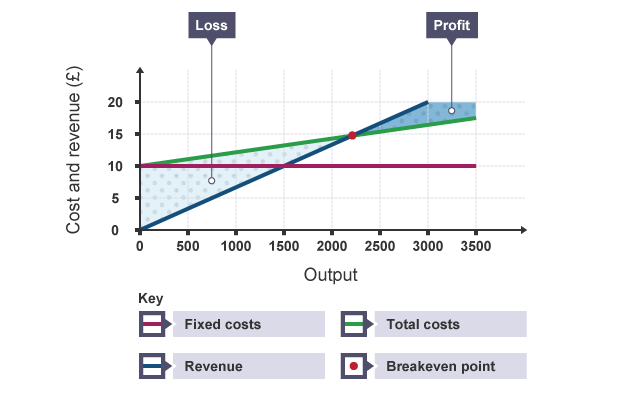?

## Break Even

Break even is the point when a businesses profits are equal to the costs and the business is not making a profit or a loss.

1 of 11

## Break Even Chart2 of 11

## Revenue

Revenue is the income earned by a business over a period of time. The amount of revenue earned depends on two things - the number of items sold and their selling price.

revenue = price x quantity

Revenue is sometimes called sales, sales revenue, total revenue or turnover.

3 of 11

## Costs

Costs are the expenses involved in making a product. Firms incur costs by trading.

4 of 11

## Variable cost

Some costs, called variable costs, change with the amount produced. For example, the cost of raw materials rises as more output is made.

5 of 11

## Fixed costs

Other costs, called fixed costs, stay the same even if more is produced. Office rent is an example of a fixed cost which remains the same each month even if output rises.

6 of 11

## Total costs

The total cost is the amount of money spent by a firm on producing a given level of output. Total costs are made up of fixed costs (FC) and variable costs (VC).

Fixed costs + Variable costs

7 of 11

## Profit

Profit is the surplus left from revenue after paying all costs. Profit is found by deducting total costs from revenue.

profit = total revenue - total costs.

8 of 11

## Losses

A loss is made when the revenue from sales is not enough to cover all the costs of production.

9 of 11

## Gross Profit

Gross profit is the difference in value between the revenue generated by a product or service and the cost of producing it. The latter is commonly known as 'cost of sales' or 'direct costs', and generally includes things such as materials, distribution costs and labour costs.In other words, gross profit represents the amount of value gained from the sale of a product or service.

10 of 11

11 of 11